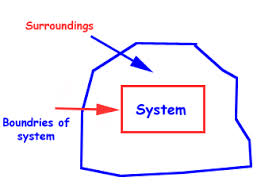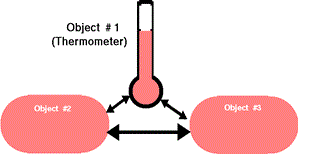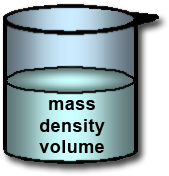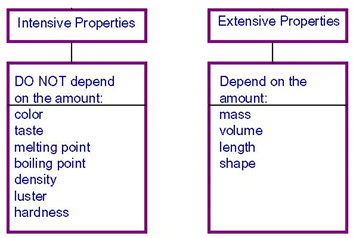×#### Thank you for registering.

One of our academic counsellors will contact you within 1 working day.

Click to Chat

1800-1023-196

+91-120-4616500

CART 0

• 0

MY CART (5)

Use Coupon: CART20 and get 20% off on all online Study Material

ITEM
DETAILS
MRP
DISCOUNT
FINAL PRICE
Total Price: Rs.

There are no items in this cart.
Continue Shopping• Complete Physics Course - Class 11
• OFFERED PRICE: Rs. 2,968
• View Details

```Thermodynamic State of a System and Macroscopic Intensive, Extensive Properties

Table of Content

Thermodynamics

Thermal Equilibrium and Concept of Temperature [Zeroth Law of Thermodynamics]

Equation of State

Equilibrium of a System

Macroscopic Properties of a System

Extensive Properties

Intensive Properties

Solved Problems

Related Resources

Thermodynamics“It is the branch of physics which deals with process involving heat, work and internal energy. Thermodynamics is concerned with macroscopic behavior rather than microscopic behavior of the system.”

A thermodynamic system is one which can be described in terms of the thermodynamic co-ordinates. The co-ordinates of a thermodynamic system can be specified by any pair of quantities like pressure (P), volume (V), temperature (T), and entropy (S). The thermodynamic systems in engineering are gas, vapour, steam, mixture of gasoline vapour and air, ammonia vapours and its liquid. In Physics, thermodynamics includes besides the above systems, like stretched wires, thermocouples, magnetic materials, electric condenser, electrical cells, solids and surface films.“A thermodynamic state is the macroscopic condition of a thermodynamic system as described by its particular thermodynamic parameters. The state of any thermodynamic system can be described by a set of thermodynamic parameters, such as temperature, pressure, density, composition, independently of its surroundings or history.”

Example 1:-

Stretched Wire:- In a stretched wire, to find the Young’s modulus of a wire by stretching, the complete thermodynamic co-ordinates are

(a) the stretching force F

(b) the length of the stretching wire and

(c) the temperature of the wire

The pressure and volume are considered to be constant

Example 2:-

Surface Films:- For liquid films, in the study of surface tension, the thermodynamic co-ordinates are

(a) the surface tension

(b) the area of the film and

(c) the temperature

Example 3:-

Reversible Cells:- The thermodynamic co-ordinates to completely describe a reversible cell are

(a) the E.M.F of the cell

(b) the charge that flows and

(c) the temperature

Thermal Equilibrium and Concept of Temperature [Zeroth Law of Thermodynamics]A thermodynamic system is said to be in thermal equilibrium if any two of its independent thermodynamic co-ordinates X and Y remain constant as long as the external conditions remain unaltered. Consider a gas enclosed in a cylinder fitted with a piston. If the pressure and volume of the enclosed mass of gas are P and V at the temperature of the surroundings, these values of P and V will remain constant as long as the external conditions viz. temperature and pressure remain unaltered. The gas is said to be in thermal equilibrium with the surroundings.

The zeroth law of thermodynamics was formulated after the first and second laws of thermodynamics have been enunciated. This law helps to define the term temperature of a system.

This law states that,

“if of three systems, A, B and C, A and B are separately in thermal equilibrium with C, then A and B are also in thermal equilibrium with each other”.Conversely the law can be stated as follows:-

“If three or more systems are in thermal contact, each to each, by means of diathermal walls and are all in thermal equilibrium together, then any two systems taken separately are in thermal equilibrium with one another”.

Therefore, the temperature of a system can be defined as the property that determines whether or not the body is in thermal equilibrium with the neighboring systems. If a number of systems are in thermal equilibrium, this common property of the system can be represented by a single numerical value called the temperature. It means that if two systems are not in thermal equilibrium , they are at different temperatures.

Example:-

In a mercury in glass thermometer, the pressure above the mercury column is zero and volume of mercury measures the temperature. If a thermometer shows a constant reading in two systems A and B separately, it will show the same reading even when A and B are brought in contact.

Equation of State

A relation between the values of any of the three thermodynamic variables for the system, is called its equation of state.

Equation of state for an ideal gas is PV = RT

Equilibrium of a System

A system is said to be in equilibrium if its macroscopic quantities do not change with time.

Macroscopic Properties of a SystemThe properties which are associated with a macroscopic system (i.e., having a large number of particles) are known as macroscopic properties of a system. For example, pressure, volume, temperature, composition, density, viscosity, surface tension, refractive index, colour, etc. are all macroscopic properties.

Types of properties of a system:-

Measurable properties of a system may be divided into the following two classes:

(a) Extensive Properties

These are the properties whose values depend upon the mass of the portion of the system or one can say that extensive properties depend upon the size of the system.

For example, volume, number of moles, mass, energy, internal energy, etc., are extensive properties. The volume of ten kg of matter is certainly ten times the volume of one kg of the same matter under identical conditions and so volume is an extensive property. The value of extensive property is equal to sum of the separate parts into which the system may be subdivided. Suppose Y be a property of the system A and y1,y2 and y3 be the properties of the respective subsystems a1, a2 and a3 of a system. Now Y will be an extensive property provided Y = y1+y2+y3.

(b) Intensive Properties

These are the properties of a system whose values are independent of the quantity of matter contained in the system. If a system is divided into n parts, each part has the same value of the intensive property. Thus, the intensive properties are characteristic of the substances present in the system. Such properties do not require any specification of the size of sample to which they refer. For example, refractive index, viscosity, surface tension, density, temperature etc., are all intensive properties. Whether we consider one kg or ten kg of a given system at uniform temperature, its refractive index remains the same in both the cases, and so refractive index is regarded as an intensive property.Temperature is an intensive property because if we take 1000 ml water in a beaker at room temperature and divide this into ten equal parts (100 ml each) or nine equal parts (500 ml, 250 ml, 100 ml, 50 ml, 30 ml, 25 ml, 20 ml, 15 ml and 10 ml) or in any other proportion, the temperature of each part will remain the same.

Mole fraction is regarded as an intensive property because once homogenous mixture is prepared, the relative amount of the constituents remain same in every part of the mixture.

Molarity is regarded as an intensive property because it remains same for each part of the homogeneous mixture. On the other hand, the number of moles is an extensive property because the total number of moles of system will be the sum of the moles of the each subsystem.The extensive and intensive properties have been given in below table:

Extensive Property

Intensive Property

Volume

No of moles

Mass

Energy

Free energy

Entropy

Enthalpy

Heat capacity

Molar volume

Density

Refractive index

Surface tension

Viscosity

Dielectric constant

Molar enthalpy

Molar entropy

Chemical potential

Free energy per mole

Specific heat

Pressure

Temperature

Boiling point, Freezing point, etc.The extensive properties of a single pure substance not only depend upon the number of moles (n) of the substance present but also on any two of three variables P, V, and T, called independent variables.

The product, ratio and sum of any intensive properties are always intensive.

If any extensive property is expressed per mole or per gram, it becomes an intensive property.

A thermodynamic system is a collection of matter which has distinct boundaries.

A thermodynamic system is that part of universe which is under thermodynamic study.

Solved Problems

Problem 1:-

What mass of system at 100ºC must be mixed with 150 g of ice at 0ºC, in a thermally insulated container, to produce liquid water at 50ºC?

Concept:-

The heat capacity per unit mass of a body, called specific heat capacity or usually just specific heat, is characteristic of the material of which the body is composed.

c = C/m

= Q/mΔT

So, Q = c mΔT

Here, the heat transferred is Q, specific heat capacity is c, mass is m and the temperature difference is ΔT.

The amount of heat per unit mass that must be transferred to produce a phase change is called the heat of transformation or latent heat L for the process. The total heat Q transferred in a phase change is then,

Q = Lm

Here m is the mass of the sample that changes phase.

Solution:-

The heat given off the steam Qs will be equal to,

Qs = msLv+ mscwΔT

Here, mass of steam is ms, latent heat vaporization is Lv, specific heat capacity of water is cw and the temperature difference is ΔT.

The heat taken in by the ice Qi will be equal to,

Qi = miLf+ micwΔT

Here, mass of ice is mi, latent heat fusion is Lf, specific heat capacity of water is cw and the temperature difference is ΔT.

Heat given off the steam Qs is equal to the heat taken in by the ice Qi.

So, Qs = Qi

msLv+ mscwΔT = miLf+ micwΔT

ms(Lv+ cwΔT) = mi(Lf+ cwΔT)

ms = mi(Lf+ cwΔT)/ (Lv+ cwΔT)

To obtain the mass of the steam at 100 °C must be mixed with 150 g of ice at 0 °C, substitute 150 g for mass of ice mi, 333×103 J/kg for Lf, 4190 J/kg.K for cw, 50° C for ΔT, 2256×103 J/kg for Lv in the equation ms = mi(Lf+ cwΔT)/ (Lv+ cwΔT), we get,

ms = mi(Lf+ cwΔT)/ (Lv+ cwΔT)

=(150 g)[(333×103 J/kg) +(4190 J/kg.K) (50° C)]/ [(2256×103 J/kg)+ (4190 J/kg.K) (50° C)]

=(150 g×(10-3 kg/1g))[(333×103 J/kg) +(4190 J/kg.K) (50+273)K]/ [(2256×103 J/kg)+ (4190 J/kg.K) (50+273)K]

= 0.033 kg

From the above observation we conclude that, the mass of steam at 100 °C must be mixed with 150 g of ice at 0 °C would be 0.033 kg.

_______________________________________________________________________________

Problem 2:-

In a motorcycle engine, after combustion occurs in the top of the cylinder, the piston is forced down as the mixture of gaseous products undergoes an adiabatic expansion. Find the average power involved in this expansion when the engine is running at 4000 rpm, assuming that the gauge pressure immediately after combustion is 15.0 atm, the initial volume is 50.0 cm3, and the volume of the mixture at the bottom of the stroke is 250 cm3. Assume that the gases are diatomic and that the time involved in the expansion is one-half that of the total cycle.

Concept:-

In an adiabatic process, the final pressure pf is defined as,

pf = piViγ/Vfγ

= pi [Vi/Vf]γ

The work done W for adiabatic process is defined as,

W = [pfVf - piVi]/(γ-1)

Here, initial pressure is pi, initial volume is Vi and final volume is Vf and adiabatic constant is γ.

Solution:-

First we have to find out final pressure pf. To obtain pf, substitute 16.0 atm for pi, 50.0 cm3 for Vi, 250 cm3 for Vf and 1.40 for γ in the equation pf = pi [Vi/Vf]γ,

pf = pi [Vi/Vf]γ

=(16.0 atm) [50/250]1.40

= 1.68 atm

To obtain the work done W by the gas during the expansion, substitute 16.0 atm for pi, 50.0 cm3 for Vi,1.68 atm for pf, 250 cm3 for Vf and 1.40 for γ in the equation W = [pfVf - piVi]/(γ-1), we get,

W = [pfVf - piVi]/(γ-1)

= (16.0 atm) (50.0 cm3) – (1.68 atm) (250 cm3)/(1.40-1)

= [(16.0 atm×(1.01×105 Pa/1 atm)) (50.0 cm3×(10-6 m3/1 cm3))] –[ (1.68 atm×(1.01×105 Pa/1 atm)) (250 cm3×(10-6 m3/1 cm3))]/(1.40-1)

=96.0 Pa.m3

= (96.0 Pa.m3) (1 N/m2/ 1 Pa)

= (96.0 N.m) (1 J/ 1 N.m)

= 96.0 J

The above process happens 4000 times per minute, but the actual time to complete the process is half of the cycle, or 1/8000 of a minute.

Thus the average power P involved in this expansion when the engine is running at 4000 rpm will be,

P = (96.0 J) (8000)/(60 s)

= 12.8×103 J/s

= (12.8×103 J/s) (1 W/1 J/s)

= 12.8×103 W

From the above observation we conclude that, the average power P involved in this expansion when the engine is running at 4000 rpm would be 12.8×103 W.Question 1:-

The thermodynamic properties that are independent on the size of the system is called _____.

A. Extensive property

B. Intensive property

C. Open property

D. Closed property

Question 2:-

The thermodynamic properties that are dependent on the size or extent of the system is called _____.

A. Extensive property

B. Intensive property

C. Open property

D. Closed property

Question 3:-

Which is NOT an intensive property of thermodynamics?

A. Temperature

B. Mass

C. Pressure

D. Density

Question 4:-

Which is NOT an extensive property of thermodynamics?

A. Density

B. Mass

C. Volume

D. Energy

Question 5:-

Extensive properties per unit mass are called _____.

A. Specific properties

B. Relative properties

C. Unit properties

D. Phase propertiesQ.1
Q.2
Q.3
Q.4
Q.5

b
a
c

a

a

Related Resources

You might like to refer Thermal Physics.

For getting an idea of the type of questions asked, refer the  Previous Year Question Papers.

To read more, Buy study materials of Thermodynamics comprising study notes, revision notes, video lectures, previous year solved questions etc. Also browse for more study materials on Chemistry here.
```### Course Features

• 101 Video Lectures
• Revision Notes
• Previous Year Papers
• Mind Map
• Study Planner
• NCERT Solutions
• Discussion Forum
• Test paper with Video Solution